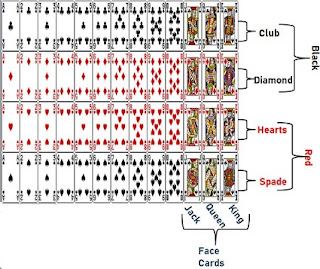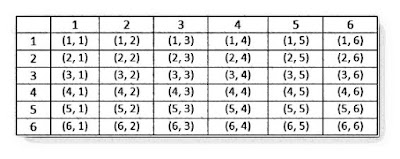## Revision Notes for Ch 15 Probability Class 10th Mathematics

Basic Terms

• Probability: Probability is a concept which numerically measures degree of certainty of the occurrence of events.

• Experiment: An operation which can produce some well-defined outcomes is called an experiment.

• Random Experiment: An experiment in which all possible outcomes are known.

• Trial: means performing a random experiment.

Probability of Occurrence of an EventFor example, what is the probability of the getting head, when we toss the coin.

Possible Outcomes when we toss a coin: Head or Tail
So, we have two possible outcomes.
So, number of possible outcomes =2
So, we have only one favorable outcome
Probability is given asExample of Experiment: Tossing A CoinIf we toss a coin, it would be head or tail, only two outcomes.

Example of Experiment: Tossing A DiceA dice has six outcomes, numbering 1 to 6.

Example of Experiment: Deck of Cards

A deck of playing cards contains 52 Cards.If we pick any card from the deck, the outcome will be any one card out of these 52.
There are 13 sets of same type of card. The 4 types of cards are Club, diamond, hearts and Spade. Heart and spade are red in color, while club and diamond are black in color.

So, there are 13 club, 13 spade, total 26 Black Cards; and 13 hearts, 13 spade total of 26 red cards.

There are 3 face cards in each set.
Jack, Queen and king.

So, there are 3 face cards on each of the 4 sets. So, there are 12 face cards.
Also, we can say there are 6 black face cards, and 6 red face cards.

Apart from face cards, other cards are A, and cards numbering 2 to 10.

Example of Experiment: Tossing two diceNow, there will be 6 outcomes of each dice, multiplied we will get 6×6 = 62 =36 Outcomes. They are: